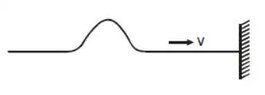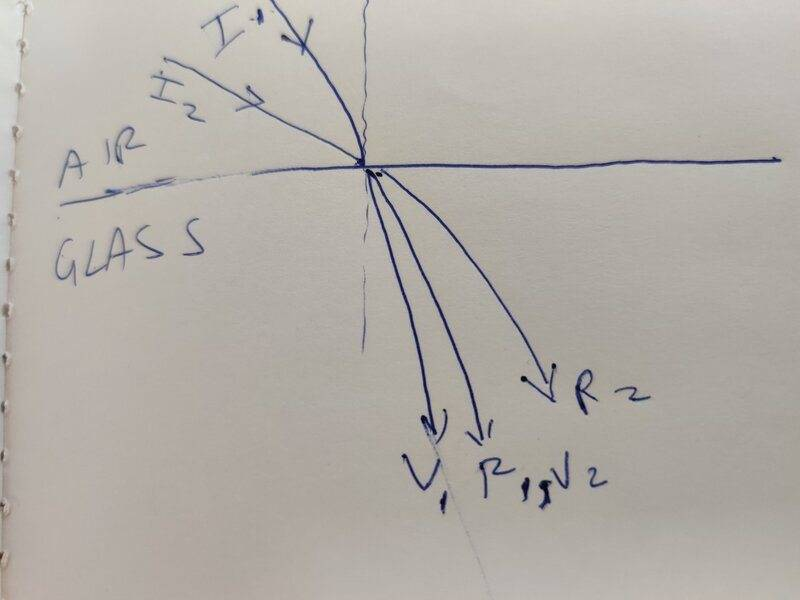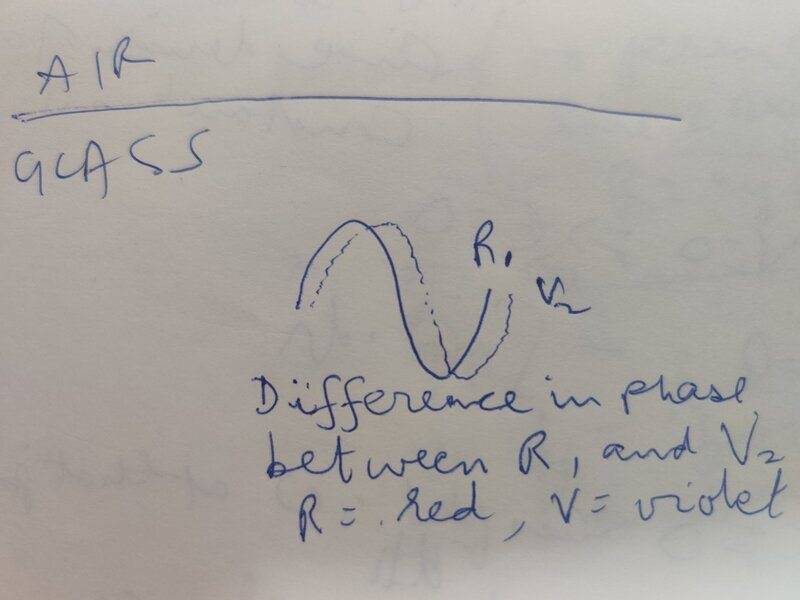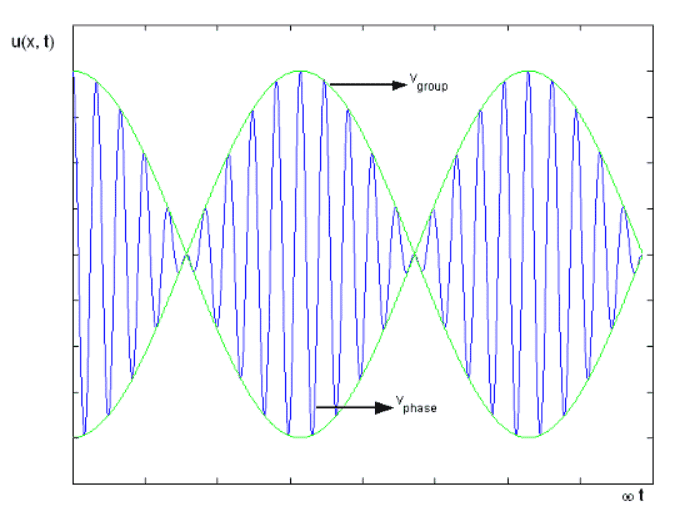# Why does a traveling wave pulse get distorted?

• vcsharp2003
vcsharp2003
Homework Statement
When a pulse passes through a dispersive medium then why does it get distorted in the medium?
Relevant Equations
None
I am getting confused by this question. Nevertheless, I tried answering this question.

When I see the word pulse, it brings to my mind a pulse traveling in a rope as shown in diagram below and I cannot relate dispersion to the rope medium in which pulse is travelling. What I do know is that white light gets dispersed as it passes through another medium like glass due to different refractive index for each color in the incident white light resulting in different angles of refraction in glass for different colors of light. But I cannot see any distortion occurring in incident sinusoidal light wave since the refracted light for each color is also a sinusoidal wave.1. Can I have a pulse of light or pulse of sound? Probably not. I can relate a wave to sound or light as in a sinusoidal wave.
2. Even though light is getting dispersed in glass, how is the light wave getting distorted? The light wave for each color in glass is still a sinusoidal wave and I cannot see where the distortion is.
3. Does the word pulse in this question mean a sinusoidal wave? To me, pulse means what is shown in the diagram above.
4. Can there be dispersion of a pulse in a rope? Probably not, since the pulse keeps traveling in a rope unchanged until it hits a fixed/free boundary where reflection occurs. If the boundary was a free boundary, then a part of the pulse gets reflected and a part gets transmitted with a lesser amplitude.

vcsharp2003
Homework Statement:: When a pulse passes through a dispersive medium then why does it get distorted in the medium?
Relevant Equations:: None

Can I have a pulse of light or pulse of sound? Probably not. I can relate a wave to sound or light as in a sinusoidal wave.
I think if the source of light or sound wave is on for a few moments and then turned off, it will send a pulse of light or sound through a medium. A wave on the other hand would mean that the source of disturbance is continuously operating and continuously sending out disturbance.

So, there could be a pulse of light or sound.

Homework Helper
Gold Member
2022 Award
Homework Statement:: When a pulse passes through a dispersive medium then why does it get distorted in the medium?
Relevant Equations:: None

Even though light is getting dispersed in glass, how is the light wave getting distorted? The light wave for each color in glass is still a sinusoidal wave and I cannot see where the distortion is.
If you have a sinusoidal wave of light of multiple frequencies in which each frequency has sinusoidal amplitude, and these wave forms are all in phase, then the dispersive medium will separate them somewhat. Will the sum of such separated sinusoids still be sinusoidal?

•vcsharp2003
vcsharp2003
If you have a sinusoidal wave of light of multiple frequencies in which each frequency has sinusoidal amplitude, and these wave forms are all in phase, then the dispersive medium will separate them somewhat. Will the sum of such separated sinusoids still be sinusoidal?
Yes, the sum of sinusoid for each color will still be a sinusoid since these color waves are in phase. This summation happens in incident white light and not in dispersed medium. I am thinking that separated/dispersed light waves of different colors cannot interfere and I am not sure if that's right?

After dispersion, each separated sinusoid will be a separate wave and therefore, they will not result in wave interference. But the incident white light will be a result of interference of in-phase multiple sinusoids that will cause the resulting wave to have a larger amplitude.

Last edited:
Staff Emeritus
Homework Helper
Gold Member
While light can many times be approximated by a sinusoidal wave, a true sinusoidal wave goes on forever and the typical light signal does not. This effectively means that the wave is not composed out of a single frequency, but rather a spectrum of different frequencies (the mathematics describing this is the Fourier transform). The distortion of a pulse comes from different frequencies traveling at different speeds and therefore leading to a different interference pattern among the frequencies. So to summarize:

1. Yes. Unless you have a sound or light that goes on forever, it will not be perfectly sinusoidal although this may be a good approximation in many cases.

2. The different colors represent different frequencies of light. The different frequencies travel at different speeds due to different refractive index and therefore the interference pattern between the colors changes.

3. No, it does not.

4. Yes and no. At the most basic description, the wave velocity in the rope depends only on the rope's tension and linear density (and not wave frequency), which means all frequencies travel at the same speed. Because of this, there is no dispersion. If you go to higher order descriptions, there may be sub-leading effects that result in dispersion, but at leading order the pulse will retain its shape.

•vcsharp2003
vcsharp2003
Yes and no. At the most basic description, the wave velocity in the rope depends only on the rope's tension and linear density (and not wave frequency), which means all frequencies travel at the same speed. Because of this, there is no dispersion. If you go to higher order descriptions, there may be sub-leading effects that result in dispersion, but at leading order the pulse will retain its shape.
If the rope is not uniform in its mass density, then the same pulse will change its velocity as it travels through the rope. But then how will the pulse disperse in rope?

Staff Emeritus
Homework Helper
Gold Member
If the rope does not have a uniform mass density this will introduce non-linearities. The wave equation will no longer have constant coefficients. You will introduce effects such as wave reflection where you have changes in velocity. This can become quite messy.

•vcsharp2003
vcsharp2003

The different frequencies travel at different speeds due to different refractive index and therefore the interference pattern between the colors changes.
Why would separated colors interfere? My impression is that each color would have vibrations about different paths and so they would not interfere.

Or we need to consider multiple incident rays of white light at different angles, and then refraction of colors of these different incident white light rays could result in different colors traveling along the same path and therefore, having a superposition of color waves?Last edited:
vcsharp2003
Will the sum of such separated sinusoids still be sinusoidal?
No, if the different color waves follow the same path in refractive medium since being on same path means they will superimpose and also they will have a certain non-zero path difference or phase difference due to different velocities in glass. The second diagram show red light and violet light in glass that come from incident waves ##I_1## and ##I_2## respectively.Last edited:
Homework Helper
Gold Member
2022 Award
they will have a certain non-zero path difference or phase difference
I think you are confusing two concepts. We are not concerned with phase difference in light as a wave.
A pulse of light can not only consist of many frequencies but also be many wavelengths long of each frequency. A shift in phase of some frequencies relative to others might not be evident, but if one frequency travels fast than another the entire train of waves of that frequency will arrive earlier.
See group velocity dispersion at https://en.m.wikipedia.org/wiki/Dispersion_(optics).

•vcsharp2003
Gold Member
Homework Statement:: When a pulse passes through a dispersive medium then why does it get distorted in the medium?
Relevant Equations:: None

I am getting confused by this question. Nevertheless, I tried answering this question.

When I see the word pulse, it brings to my mind a pulse traveling in a rope as shown in diagram below and I cannot relate dispersion to the rope medium in which pulse is travelling.
You need to go back to basics as it will be more efficient. What do you suppose causes dispersion in the first place? Yes, it occurs because different wavelengths travel at different speeds, but what causes that? To understand that you need to look at how a wave makes its way through a medium.

Homework Helper
Gold Member
2022 Award
You need to go back to basics as it will be more efficient. What do you suppose causes dispersion in the first place? Yes, it occurs because different wavelengths travel at different speeds, but what causes that? To understand that you need to look at how a wave makes its way through a medium.
That seems to me to be beyond the expectations of the question. It provides that the medium is dispersive, and we can take that as meaning the frequencies travel at different rates. We are only asked to explain how that leads to distortion of the pulse. Why they travel at different rates is another question.

•vcsharp2003
vcsharp2003
I think you are confusing two concepts. We are not concerned with phase difference in light as a wave.
A pulse of light can not only consist of many frequencies but also be many wavelengths long of each frequency. A shift in phase of some frequencies relative to others might not be evident, but if one frequency travels fast than another the entire train of waves of that frequency will arrive earlier.
See group velocity dispersion at https://en.m.wikipedia.org/wiki/Dispersion_(optics).
I need to first understand the concepts of group velocity and phase velocity. The wave velocity that I know is like the phase velocity.

I came across this link http://Group velocity and Phase velocity which was easier to understand for these concepts. I now get what group velocity means versus phase velocity.

Below is a diagram that is the resultant wave of superposition of two different frequencies. We see a symmetrical green line i.e. symmetrical envelope.At the link I mentioned, it talks about dispersion in the context of above diagram.
If the derivative term is zero, group velocity equals phase velocity. In this case, there is no dispersion. Dispersion is when the distinct phase velocities of the components of the envelope cause the wave packet to "spread out" over time. The components of the wave packet (or envelope) move apart to the degree where they no longer combine to complete the envelope.
The wave packet seems to be what's within a peak or crest of the green line. When velocities of component waves/pulses change from the values that produced the envelope (i.e. green line) then the superposition of new waves/pulses changes the envelope i.e. green line. The envelope could now become asymmetrical and there is no proper identification of a wave packet. Is this what you meant, when you said "Will the sum of such separated sinusoids still be sinusoidal?"?

Last edited:
vcsharp2003
I think you are confusing two concepts. We are not concerned with phase difference in light as a wave
You meant that there could be no change in the original phase difference between the individual waves/pulses but only a change in their original velocities and this change in velocities would result in separation of these waves/pulses after a certain time of propagation, causing a superposition of them that's going to look different from their original superposition.

Last edited:
Gold Member
I think a fuller explanation of the question and its context are needed.

Being of the Electronic persuasion, I took "Pulse" to mean a electrical signal with fast rise & fall times (a pulse) in a dispersive medium. Of course it could also be seismic waves in the Earth, or Water waves in the Ocean from an underwater landslide or volcanic eruption.

The explanation of pulse distortion in this context is rather straight-forward.

Cheers,
Tom

Homework Helper
Gold Member
2022 Award
I need to first understand the concepts of group velocity and phase velocity. The wave velocity that I know is like the phase velocity.

I came across this link http://Group velocity and Phase velocity which was easier to understand for these concepts. I now get what group velocity means versus phase velocity.

Below is a diagram that is the resultant wave of superposition of two different frequencies. We see a symmetrical green line i.e. symmetrical envelope.

View attachment 298384

At the link I mentioned, it talks about dispersion in the context of above diagram.

The wave packet seems to be what's within a peak or crest of the green line. When velocities of component waves/pulses change from the values that produced the envelope (i.e. green line) then the superposition of new waves/pulses changes the envelope i.e. green line. The envelope could now become asymmetrical and there is no proper identification of a wave packet. Is this what you meant, when you said "Will the sum of such separated sinusoids still be sinusoidal?"?
Yes. In the diagram above, a pulse is one rise and fall of amplitude, i.e. the upper green line between two zeroes. It could be any single-peaked shape.
If a pulse is the sum of such with different frequencies then dispersion will cause them to separate, changing the shape of the pulse as a whole.
You meant that there could be no change in the original phase difference between the individual waves/pulses
Not sure what you mean by that. I'm saying that phase at the level of the blue line in your diagram is not relevant, and has no equivalent in some other pulse contexts.

•vcsharp2003
vcsharp2003
Not sure what you mean by that. I'm saying that phase at the level of the blue line in your diagram is not relevant,
What I meant is explained below in the context of blue/green line diagram.

The blue line wave line is formed by the superposition of two waves that differ slightly in frequency (as mentioned at the link Group velocity vs phase velocity). These two component waves can have no phase difference i.e. the ##\phi## part in their respective wave equations or a constant phase difference. Now phase difference between waves is what makes the peak of one be offset/separated from the nearest peak of the other. However, if now their velocities change so that this offset/separation between component waves changes then the superposition of these waves will produce a different envelope i.e. green line. So, in this scenario the component waves have maintained their phases but their velocities have changed.

•